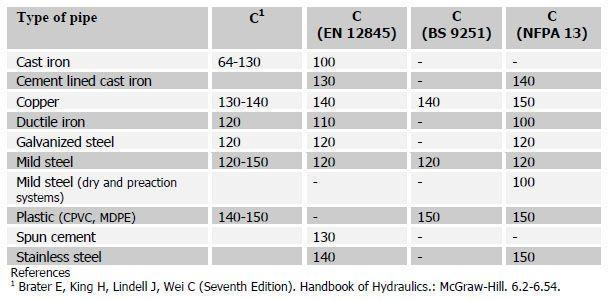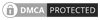## Hazen Williams formula for use in fire sprinkler systems

The Hazen Williams formula is an empirical equation and has long been used for calculating the friction loss in pipework for water based fire sprinkler protection systems. This equation uses the coefficient C to specify the pipes roughness, which is not based on a function of the Reynolds number, as in other pressure loss equations. This however has the disadvantage that the equation can only be used when water is flowing within the 'turbulent' flow range. If the system is outside the normal pressure and flow range or the system is to use additives, or will be subject to unusual temperature conditions then the Darcy Weisbach equation may be more appropriate.

Gardner Williams and Allen Hazen first started there Experimentation in the early part of the 20th century.  They began to record the friction loss through pipe in numerous experimentations in their studies resulted in the development of a empirical formula which we know today as the Hazen- Williams formula.  The formula along with a series of friction loss tables computed from the formula was published in 1903.

The Hazen Williams formula has the advantage of been simple to calculate by using a scientific calculator where as the Darcy Weisbach equation requires the use 'f' friction factor and this can only be calculated by an number of iterations as 'f' is on both sides of the equation. You can use a Moody diagram to find the value of 'f' however this is both time consuming and almost certainly not an inaccurate method.

The Hazen William formula has now become adopted through the world as the pressure loss formula to use for the hydraulic design of fire sprinkler systems and in almost all cases the use of the hazen william formula will provide adequate answers. The Hazen William formula can also be used for the calculation of water mist systems where the system pressure does not exceed 12 bar (low pressure water mist systems) or the water velocity does not exceed 7.6 m/s and the minimum pipe size is 20mm in the case of intermediate and high pressure water mist systems.

You can use Canute Hcalc hydraulic calculator or our online Hazen Williams calculator to visually explore the relationship between the flow, pipe diameter and the pipe C-Factor in the Hazen Williams formula which will give you a good understanding of the formula. The Hcalc software is free to download and use.when:

p = pressure loss in bar per meter
Q = flow through the pipe in L/min
C = friction loss coefficient
d = internal diameter of the pipe in mm

You can seen the above equation that if Q is raised to the power of 1.85 in the above equation this has the effect that if the flow is doubled and all other things remain constant the friction loss will increase by almost 4 times, if the flow was to triple the friction loss would almost be 9 times greater.  You can also see that the pipe diameter D is raised to the power of 4.87 and that it's in the denominator on the right hand side of the equation.  Therefore any increase in the pipe size will reduce the friction loss if all other factors remain the same.  If the diameters doubled, the friction loss will be reduced by almost a factor of 1/32 likewise if the pipe diameter is tripled The friction loss would be reduced to about 1/243 of its original value.

The Hayes-Williams formula which is empirical yields only approximate results however it is considered to be accurate enough to be used for the calculation of fire sprinkler systems and indeed the formula is stipulated in both NFPA 13 and EN 12845.  There are certain cases such as high pressure water mist where the use of Darcy-Weigbach formula would be more appropriate and this is set out in NFPA 750.

## Value of C for use in the Hazen-Williams formula

Listed in the table bellow are typical values for the coefficient C, which can be used in the Hazen-Williams formula for different fire sprinkler design standards. The value of C represents the pipes roughness with higher values of C giving lower friction losses. The values given in the design standards allow for degradation of the pipe, for instance new cast iron pipe has a C coefficient of 130 and EN 12845 gives the value of 100, this is equivalent to a pipe, which is about 20 years oldCanute LLP
15 Queen Square
Leeds, West Yorkshire
United Kingdom LS2 8AJ

t: +44 (0) 113 328 0350
e: info@canutesoft.com

 Copyright © 2021, Canute LLPRegistered in England & Wales, Partnership No. OC305985